×#### Thank you for registering.

One of our academic counsellors will contact you within 1 working day.

Click to Chat

1800-1023-196

+91-120-4616500

CART 0

• 0

MY CART (5)

Use Coupon: CART20 and get 20% off on all online Study Material

ITEM
DETAILS
MRP
DISCOUNT
FINAL PRICE
Total Price: Rs.

There are no items in this cart.
Continue Shopping```Sections of a Cone

Table of Content

Meaning of Cone

How Cone is formed?

What is double napped right circular Cone?

Why is it called right circular cone?

Cross Sections of a Cone

Conic Sections

What are the four types of conic sections?

Why is it called a conic section?

Why are conics important?

Sections of a Cone

Degenerate conics

How is a circle formed?

Surface Area of a Cone

Surface Area of a Cone Section

Volume of a Cone

Volume of Cone Section

Cone Section calculator

Meaning of Cone

Cone is a 3D shape which has flat base and round lateral surface with a point on top. The point is called Apex or Vertex. The things having cone shape are called Conical.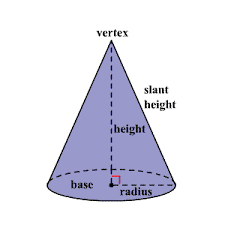How Cone is formed?

Cone is basically a formed by a rotated triangle. In a right angled triangle, its longer side rotates around its shorter side. So that it forms a cone. The side it rotates around is called axis of the cone. As the height shown in the above picture is the axis.

What is double napped right circular Cone?

When there are two lines intersect each other at a fixed point and at an angle  in which one is fixed vertical line .when the line rotates around the fixed vertical line so that the angle remains same, we got a double napped right circular cone. Nappe is the lateral surface or the round surface of the cone. The cone above the vertex is called Upper Nappe and the cone below the vertex is called Lower Nappe.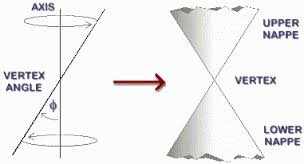The fixed vertical line here is called the Axis.

The line which is rotating is called the Generator.

The fixed angle is called Vertex Angle.

The intersection point of two lines is called Vertex.

The vertex divides the cone in two nappes.

Why is it called right circular cone?

It is called circular as the base is circle and right because the axis is perpendicular to the centre of the base to the plane. And in other words, it is made by the rotated right angled triangle so it is called right circular cone.

Cross Sections of a Cone

When we cut an object into slices with a plane we get so many parallel cross sections. Likewise if we cut a right circular cone in pieces we could get different cross sections from it depending upon the way we cut the cone.

The possible cross sections from a cone are Circle, Ellipse, Parabola and Hyperbola.

Conic Sections

If we intersect a plane with the right circular cone, we get a section, and that particular section is called a conic section. Or you can say that if we slice a 3D cone with a plane from different angles then we will get different sections and these sections are called the conic sections.

In German it is called Kegelschnitt.

What are the four types of conic sections?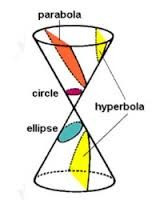Conic Section is a curve formed by the intersection of a plane with the two napped right circular cone.

There are four types of conic sections, like, circle, ellipse, parabola and hyper bola.

Why is it called a conic section?

The circle, ellipse, parabola and hyperbola are called the conic sections because these are formed by the intersection of a plane with the cone. “Conic” as these are made out of a right circular cone and “section” as these are the sections or the pieces of a cone.

Why are conics important?

Conics are important because it provide simple and useful mathematical relations. It appears in nature frequently in many ways. It has importance in our day to day life also. Generally it is used in physics as –

To study about light, we have to study about parabolas. Like if you spot the light on the parabolic mirror then all light will focus on one point, which is called Focus.

Circle is very commonly used everywhere.

The paths which you see around the sun are the ellipse having sun at focus.

In the systems of telescopes we use the hyperbolic and parabolic mirrors.

The route of objects shot near the earth’s surface usually follows a parabolic path.

To design the car headlights also, the parabola is used.

All the conics play important role in physical processes in nature. from the universe to the atom, conic sections are important.

Sections of a Cone

It depends upon the location of the plane with reference to the cone and the angle made by the vertical axis and the intersecting plane, that what kind of conic section we get.Let the angle between intersecting plane and the vertical axis be ∅.

If the plane cuts the one nappe of the cone, such that,

Ɵ < ∅ < 90°, when the plane intersects with the double circular cone in such a way that the angle between the axis and the plane is greater than the vertex angle then it made an ellipse.

∅ = 90°, when the plane is perpendicular at the axis, or the angle of intersection of plane and the vertical axis is equal to 90°, the ellipse becomes the circle. Or you can say that circle is the special type of ellipse, the section is circle.

Ɵ = ∅, when the angle between the vertical axis and the plane is equal to the vertex angle, we get an open curve called Parabola at the intersecting surface of the cone.

If the plane cuts the two nappes of the cone, such that,

Ɵ < ∅, when the angle between the plane and the vertical axis is less than the vertical angle, the plane intersects both the nappes of the cone which form two disjoint open curves are called Hyperbola.

Degenerate conics

If a plane intersects the double right circular cone at the vertex of the cone, such that

When Ɵ < ∅ < 90° in case of ellipse or ∅ = 90° in case of a circle, then the section is a point .

When Ɵ = ∅, in case of parabola, the plane contains a generator of the cone and the section is a straight line.

When 0 ≤ Ɵ < ∅, in case of hyperbola, the section is a pair of intersecting straight lines
How is a circle formed?

A circle is formed when we cut the right circular cone with a plane so that the plane is perpendicular to the symmetric axis of the cone. The curve we get with this intersection is called Closed Curve.

For circle we can say that circle is the set of all the points which are at equal distance from the center.

To calculate the coordinates of the circle to draw it in a graph we use the equation of circle-

The standard form of equation of circle is-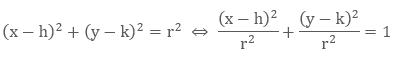where (h, k) are the coordinates of the center point and r is the radius of the circle.

If the center point (h, k) is (0, 0) then the simplified equation of circle is –

x2 + y2 = r2

The general form of equation is-

x2 + y2 + Dx + Ey + F = 0

By using these equations we can draw a circle on the graph.

We have to draw a circle with center (3,-2) and radius 4.

The equation in the standard form will be –

(x – 3)2 + (y – (-2))2 = (4)2

The circle will be like this-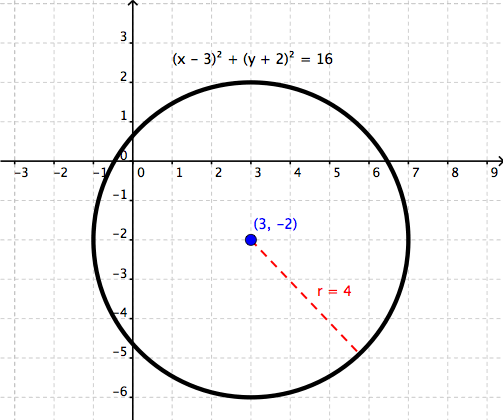Surface Area of a Cone

First we must know the height, slant length of the cone and the radius of the circle of the base. The value of π = 3.14The Surface Area of a Cone = Base Area + Curved or Lateral Surface Area

= πr2 + πrl

Surface Area of a Cone Section

A cone does not have uniform cross sections when it intersects with the plane. So the surface area will change according to the sections of the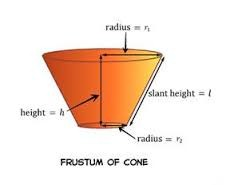cone.

In case of circle it will be called frustum. Frustum is formed when a plane cut a right circular cone in such a way that the upper and lower bases are circular and parallel.

Let the radius of the base is r1 and the radius of the top circle is r2. Height is h and the slant height as l.

The Curved Surface Area of the Section = π(r1 + r2)lVolume of a Cone

The Volume of a Cone  = 1/3πr2h

In which  is the area of the base.

R = Radius and H = height

Volume of Cone Section

Here a small cone is cut by the intersecting plane in such a way that the height is perpendicular to the center of the base of the cone.

Let R = Radius of the base of the big cone

R = Radius of the base of the small cone or the top of the frustum

H = Height of the big cone

H = Height of the small cone

To calculate the volume of the frustum we need to subtract the volume of the small cone from the volume of the big cone.

Volume of the frustum = Volume of big cone – Volume of small coneCone Section calculator

To Calculate the volume and the surface area of a cone or the sections of a cone we can use the online calculators. Like:

http://www.calculatorsoup.com/calculators/geometry-solids/cone.php

http://www.aqua-calc.com/calculate/volume-truncated-cone

Watch this Video for more reference

Sections of a Cone
```### Course Features

• 731 Video Lectures
• Revision Notes
• Previous Year Papers
• Mind Map
• Study Planner
• NCERT Solutions
• Discussion Forum
• Test paper with Video Solution